MathematicsSolving DDE Problems

This section uses an example to describe:

Example: A Straightforward Problem

This example illustrates the straightforward formulation, computation, and display of the solution of a system of DDEs with constant delays. The history is constant, which is often the case. The differential equations are

•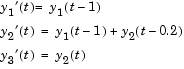The example solves the equations on [0,5] with history

•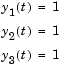for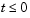.

 Note    The demo `ddex1` contains the complete code for this example. To see the code in an editor, click the example name, or type `edit ddex1` at the command line. To run the example type `ddex1` at the command line. See DDE Solver Basic Syntax for more information.

1. Rewrite the problem as a first-order system. To use `dde23`, you must rewrite the equations as an equivalent system of first-order differential equations. Do this just as you would when solving IVPs and BVPs for ODEs (see Examples: Solving Explicit ODE Problems). However, this example needs no such preparation because it already has the form of a first-order system of equations.
2. Identify the lags. The delays (lags)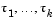are supplied to `dde23` as a vector. For the example we could use
• ```lags = [1,0.2];
```
1. In coding the differential equations,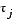= `lags(j)`.

1. Code the system of first-order DDEs. Once you represent the equations as a first-order system, and specify lags, you can code the equations as a function that `dde23` can use.
1. This code represents the system in the function, `ddex1de`.

• ```function dydt = ddex1de(t,y,Z)
ylag1 = Z(:,1);
ylag2 = Z(:,2);
dydt = [ylag1(1)
ylag1(1) + ylag2(2)
y(2)               ];
```
1. Code the history function. The history function for this example is
• ```function S = ddex1hist(t)
S = ones(3,1);
```
2. Apply the DDE solver. The example now calls `dde23` with the functions `ddex1de` and `ddex1hist`.
• ```sol = dde23(@ddex1de,lags,@ddex1hist,[0,5]);
```
1. Here the example supplies the interval of integration [0,5] directly. Because the history is constant, we could also call `dde23` by

• ```sol = dde23(@ddex1de,lags,ones(3,1),[0,5]);
```
1. View the results. Complete the example by displaying the results. `dde23` returns the mesh it selects and the solution there as fields in the solution structure `sol`. Often, these provide a smooth graph.
• ```plot(sol.x,sol.y);
title('An example of Wille'' and Baker');
xlabel('time t');
ylabel('solution y');
legend('y_1','y_2','y_3',2)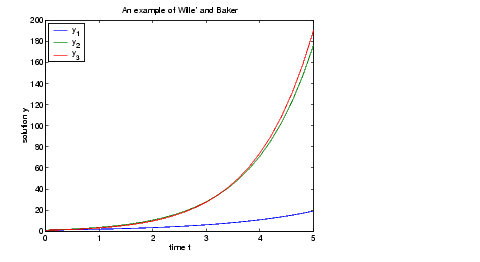```

Evaluating the Solution at Specific Points

The method implemented in `dde23` produces a continuous solution over the whole interval of integration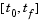. You can evaluate the approximate solution,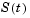, at any point inusing the helper function `deval` and the structure `sol` returned by `dde23`.

• ```Sint = deval(sol,tint)
```

The `deval` function is vectorized. For a vector `tint`, the `i`th column of `Sint` approximates the solution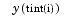.

Using the output `sol` from the previous example, this code evaluates the numerical solution at 100 equally spaced points in the interval [0,5] and plots the result.

• ```tint = linspace(0,5);
Sint = deval(sol,tint);
plot(tint,Sint);
```DDE Solver Discontinuities© 1994-2005 The MathWorks, Inc.